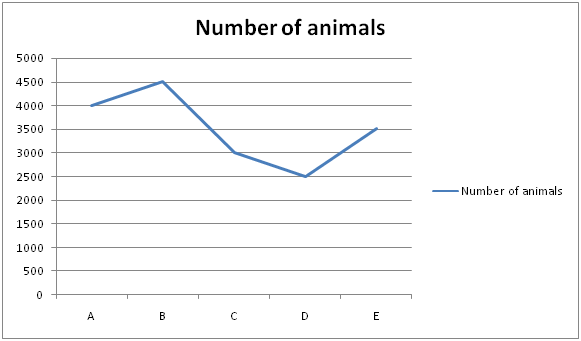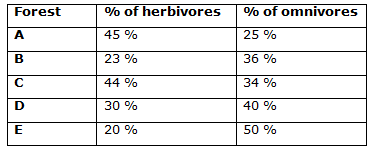# IBPS Clerk Prelims 2018 – Quantitative Aptitude Questions Day-6

Dear Readers, Bank Exam Race for the Year 2018 is already started, To enrich your preparation here we have providing new series of Practice Questions on Quantitative Aptitude – Section. Candidates those who are preparing for IBPS Clerk Prelims 2018 Exams can practice these questions daily and make your preparation effective.

[WpProQuiz 4015]

Directions (1 – 5) In the following questions, two equations I and II are given. You have to solve both the equations and give Answer as,

a) If x > y

b) If x ≥ y

c) If x < y

d) If x ≤ y

e) If x = y or the relation cannot be established

1)

I) x2 – 10x – 56 = 0

II) y2 + 27y + 72 = 0

a) a

b) b

c) c

d) d

e) e

2)

I) 2x+ 15x + 22 = 0

II) 3y2 – 2y – 16 = 0

a) a

b) b

c) c

d) d

e) e

3)

I) x2 + 17x + 52 = 0

II) y2 + 8y – 48 = 0

a) a

b) b

c) c

d) d

e) e

4)

I) 3x + 2y = 5

II) 5x – 3y = 21

a) a

b) b

c) c

d) d

e) e

5)

I) x2 + (125)1/3 = 21

II) y = ∜256

a) a

b) b

c) c

d) d

e) e

Directions (6 – 10) Study the following information carefully and answer the given questions.

The following line graph shows the number of animals in 5 different forests.The table shows the percentage of herbivores and omnivores.Note:

Number of animals = Number of Herbivores + Number of Omnivores + Number of Carnivores

Herbivores = Eating only plant

Omnivores = Eating both plant and animal

Carnivores = Eating only animal

6) Find the ratio between the total number of herbivores in forest B to that of total number of carnivores in forest E?

a) 51: 53

b) 44: 47

c) 69: 70

d) 33: 35

e) None of these

7) Find the total number of animals eating plants in forest C?

a) 2340

b) 3150

c) 2780

d) 2560

e) None of these

8) Find the difference between the total number of Omnivores in forest A and C together to that of total number of animals in forest D?

a) 360

b) 520

c) 300

d) 480

e) None of these

9) Find the average number of animals in all the given forests together?

a) 4000

b) 3500

c) 2500

d) 3000

e) None of these

10) Total number of Carnivores in forest A is what percentage of total number of Herbivores in forest D?

a) 140 %

b) 120 %

c) 160 %

d) 180 %

e) None of these

Directions (1-5) :

I) x2 – 10x – 56 = 0

(x – 14) (x + 4) = 0

X = 14, -4

II) y2 + 27y + 72 = 0

(y + 24) (y + 3) = 0

Y = -24, -3

Can’t be determined

I) 2x+ 15x + 22 = 0

2x+ 4x + 11x + 22 = 0

2x(x + 2) + 11(x + 2) = 0

(2x + 11) (x + 2) = 0

X = -11/2, -2 = -5.5, -2

II) 3y2 – 2y – 16 = 0

3y2 + 6y – 8y – 16 = 0

3y(y + 2) – 8 (y + 2) = 0

(3y – 8) (y + 2) = 0

Y = 8/3, -2

x ≤ y

I) x2 + 17x + 52 = 0

(x + 13) (x + 4) = 0

X = -13, -4

II) y2 + 8y – 48 = 0

(y + 12) (y – 4) = 0

Y = -12, 4

Can’t be determined

3x + 2y = 5 –à (1)

5x – 3y = 21 –à (2)

Solving the equation (1) and (2), we get,

X = 3, y = -2

X > y

I) x2 + (125)1/3 = 21

X2 + (53)1/3 = 21

X2 = 21 – 5

X = (16)1/2 = 4, -4

II) y = ∜256

Y = 4

x ≤ y

Directions (6-10) :

The total number of herbivores in forest B

= > 4500*(23/100) = 1035

The total number of carnivores in forest E

= > 3500*(30/100) = 1050

Required ratio = 1035: 1050 = 69: 70

The total number of animals eating plants in forest C

= > 3000*(78/100) = 2340

The total number of Omnivores in forest A and C together

= > 4000*(25/100) + 3000*(34/100)

= > 1000 + 1020 = 2020

The total number of animals in forest D = 2500

Required difference = 2500 – 2020 = 480

The average number of animals in all the given forests together

= > (4000 + 4500 + 3000 + 2500 + 3500)/5

= > 17500/5 = 3500

Total number of Carnivores in forest A

= > 4000*(30/100) = 1200

Total number of Herbivores in forest D

= > 2500*(30/100) = 750

Required % = (1200/750)*100 = 160 %

Daily Practice Test Schedule | Good Luck

 Topic Daily Publishing Time Daily News Papers & Editorials 8.00 AM Current Affairs Quiz 9.00 AM Current Affairs Quiz (Hindi) 9.30 AM IBPS PO Mains- Reasoning 10.00 AM IBPS PO Mains– Quantitative Aptitude 11.00 PM Vocabulary (Based on The Hindu) 12.00 PM IBPS PO Mains– English Language 1.00 PM IBPS PO/Clerk – GK 3.00 PM Daily Current Affairs Updates 5.00 PM IBPS Clerk Prelims – Reasoning 6.00 PM IBPS Clerk Prelims – Reasoning (Hindi) 6.30 PM IBPS Clerk Prelims – Quantitative Aptitude 7.00 PM IBPS Clerk Prelims – Quantitative Aptitude (Hindi) 7.30 PM IBPS Clerk Prelims – English Language 8.00 PM